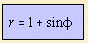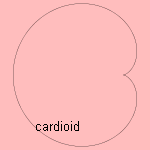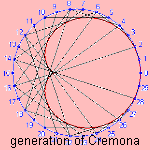# cardioid

## rouletteThis quartic 1) curve has the form of a heart 2), so that it is also called the heart curve 3). De Castillon used the name cardioid for the first time, in a paper in the Philosophical Transactions of the Royal Society (1741).
The curve is the epicycloid for which the rolling circle and the rolled circle have the same radius. Besides, the curve can be seen as a special case of the limaþon, and it is also a sinusoidal spiral.When light rays fall on a concave mirror with a large angle, no focus point but a focus line is to be seen. This line is a cardioid. The same phenomenon can occur nearby a lamp, or as a result of light falling in a cup of tea.
This seems to me the property of the cardioid that it is the catacaustic of the circle (with the source on the circumference). The cardioid is also the pedal of the circle (pedal point not on circle).

Other properties of the curve are:The cardioid is the envelope of the chords of a circle, between points P and Q, which follow the circle in the same direction, where one point has the double speed of the other. This construction is called the generation of Cremona.
This means in the figure that the points 10 and 20, 11 and 22, and so on, have been connected.Given a circle C through the origin. Then the cardioid is the envelope of the circles with as diameter the line through the origin and a point on C.

The first to study the curve was R÷mer (1674), followed by Vaumesle (1678) and KoÙrsma (1689). And more extensively by Ozanam in 1691.
La Hire found its length (4) in 1708.

Its equation can also be written as: r = cos2φ/2.
The curve can be written in a Whewell equation as s = cos φ/3 3).

notes

1) equation: (x2 +y2-y)2 = x2 +y2

2) kardia (Gr.) = heart

3) In German: Herzkurve
In Italian: cardioide.